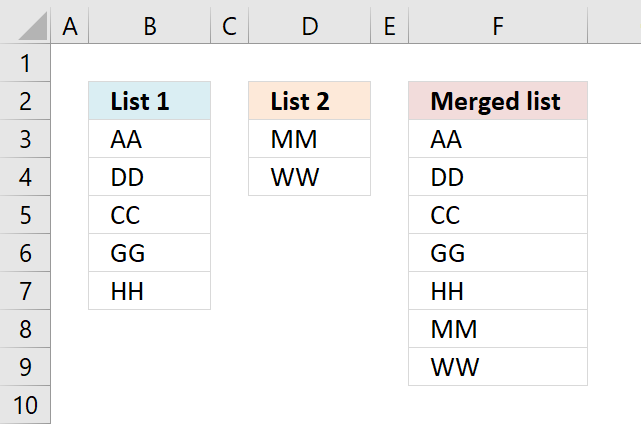Author: Oscar Cronquist Article last updated on February 05, 2018The picture above shows how to merge two columns into one list using a formula.

If you are looking for a formula to merge columns based on a condition read this article: Merge tables based on a condition

Formula in F3:

=IFERROR(INDEX(\$B\$2:\$B\$6, ROWS(C1:\$C\$1)), IFERROR(INDEX(\$D\$2:\$D\$3, ROWS(C1:\$C\$1)-ROWS(\$B\$2:\$B\$6)), ""))

Copy cell C2 and paste it down as far as needed.

Earlier versions of excel, formula in C2:

=IF(ISERROR(INDEX(\$A\$2:\$A\$6, ROWS(\$C\$1:C1))), IF(ISERROR(INDEX(\$B\$2:\$B\$3, ROWS(\$C\$1:C1)-ROWS(\$A\$2:\$A\$6))), "", INDEX(\$B\$2:\$B\$3, ROWS(\$C\$1:C1)-ROWS(\$A\$2:\$A\$6))), INDEX(\$A\$2:\$A\$6, ROWS(\$C\$1:C1)))

Copy cell C2 and paste it down as far as needed.

### How the formula in F3 works

Step 1 - Extracting List 1

=IFERROR(INDEX(\$A\$2:\$A\$6, ROWS(C1:\$C\$1)), IFERROR(INDEX(\$B\$2:\$B\$3, ROWS(C1:\$C\$1)-ROWS(\$A\$2:\$A\$6)), ""))

In cell C2: INDEX(\$A\$2:\$A\$6, ROWS(C1:\$C\$1))

becomes

INDEX(\$A\$2:\$A\$6, 1) equals "AA"

In cell C3: INDEX(\$A\$2:\$A\$6, ROWS(C1:\$C\$1))

becomes

INDEX(\$A\$2:\$A\$6, ROWS(C2:\$C\$1))

becomes

INDEX(\$A\$2:\$A\$6, 2) equals "DD"

and so on...

Step 2 - Error when all values in List 1 are processed

=IFERROR(INDEX(\$A\$2:\$A\$6, ROWS(C1:\$C\$1)), IFERROR(INDEX(\$B\$2:\$B\$3, ROWS(C1:\$C\$1)-ROWS(\$A\$2:\$A\$6)), ""))

In cell C7 something unexpected happens:

In cell C7: INDEX(\$A\$2:\$A\$6, ROWS(C1:\$C\$1))

becomes

INDEX(\$A\$2:\$A\$6, ROWS(C6:\$C\$1))

becomes

INDEX(\$A\$2:\$A\$6, 6) equals "#REF!" error

There are no more values in List 1 so we need to continue on List 2.

IFERROR() takes care of this:

IFERROR(value, value_if_error) Returns value_if_error if expression is an error and the value of the expression itself otherwise

Step 3 - Continue with List 2

In cell C7:

=IFERROR(INDEX(\$A\$2:\$A\$6, ROWS(C6:\$C\$1)), IFERROR(INDEX(\$B\$2:\$B\$3, ROWS(C6:\$C\$1)-ROWS(\$A\$2:\$A\$6)), ""))

becomes

=IFERROR(#REF!, IFERROR(INDEX(\$B\$2:\$B\$3, ROWS(C6:\$C\$1)-ROWS(\$A\$2:\$A\$6)), ""))

and becomes

IFERROR(INDEX(\$B\$2:\$B\$3, ROWS(C6:\$C\$1)-ROWS(\$A\$2:\$A\$6)), "")

INDEX(\$B\$2:\$B\$3, ROWS(C6:\$C\$1)-ROWS(\$A\$2:\$A\$6))

becomes

INDEX(\$B\$2:\$B\$3, 6-ROWS(\$A\$2:\$A\$6))

becomes

INDEX(\$B\$2:\$B\$3, 6-5)

becomes

INDEX(\$B\$2:\$B\$3, 1) equals "MM" in List 2

and so on...

Step 4 - Error when all values in List 2 and List 1 are processed

In cell C9:

=IFERROR(INDEX(\$A\$2:\$A\$6, ROWS(C8:\$C\$1)), IFERROR(INDEX(\$B\$2:\$B\$3, ROWS(C8:\$C\$1)-ROWS(\$A\$2:\$A\$6)), ""))

becomes

=IFERROR(REF!, IFERROR(INDEX(\$B\$2:\$B\$3, ROWS(C8:\$C\$1)-ROWS(\$A\$2:\$A\$6)), ""))

becomes

=IFERROR(REF!, IFERROR(INDEX(\$B\$2:\$B\$3, 8-ROWS(\$A\$2:\$A\$6)), ""))

becomes

=IFERROR(REF!, IFERROR(INDEX(\$B\$2:\$B\$3, 8-5), ""))

becomes

=IFERROR(REF!, IFERROR(REF!, "")) equals "" (nothing)